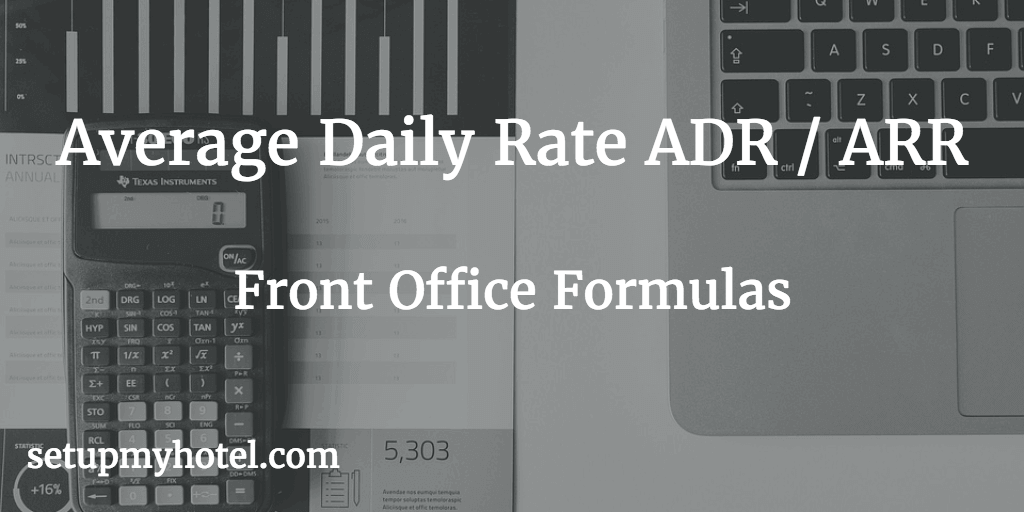Support our work by becoming our Patron! Learn More# Formula to Calculate Average Room Rate (ARR) | Average Daily Rate (ADR)

ADR (Average Daily Rate) or ARR (Average Room Rate) is a measure of the average rate paid for the rooms sold, calculated by dividing total room revenue by rooms sold.

Some hotels calculate ARR or ADR by also including the complimentary rooms this is called as Hotel Average Rate. By Taking the HARR the management can find out the actual effect of complimentary stays on the average room rate.

The formula for ARR or ADR calculation:

Average Room Rate (ARR or ADR) = Total Room Revenue / Total Rooms Sold

OR

Average Room Rate (ARR or ADR) = Total Room Revenue / Total Occupied Rooms

Average Room Rate (ARR or ADR) Calculator

Total Room Revenue :

Total Rooms Occupied:

Total Comp & House:

ARR or ADR (Occupied Rooms)=

ARR or ADR (Sold Rooms)=

Example 1:

Total Room Revenue for 01st Jan 2017   = 25000.00

Total Room Sold for 01st Jan 2017         = 250

ARR or ADR for 01st Jan 2017        = 25000.00 / 250

= 100

Example 2:

Total Room Revenue for 31st Dec 2016 = 95985.58

Total Room Sold for 31st Dec 2016       = 277

ARR or ADR for 31st Dec 2016        = 95985.58 / 277

= 346.52

Example 3 (Monthly ARR/ADR):

Total Room Revenue for the Month of October = 2,250,485.58

Total Room Sold for the Month of October       = 5822

ARR or ADR for October                             = 2,250,485.58 / 5822

= 386.55

The formula for Hotel Average Rate HARR or HADR

Average Hotel Room Rate (HARR or HADR) = Total Room Revenue / Total Rooms Sold + Comp Rooms

Example 1:

Total Room Revenue for 21st Mar 2017           = 25000

Total Room Sold for 21st Mar 2017                 = 250

Total Complimentary rooms for 21st Mar 2017 =  2

HARR or HADR for 21st Mar 2017             = 25000 / (250+2)

= 99.20

Example 2:

Total Room Revenue for 3rd Dec 2015             = 95985.58

Total Room Sold for 3rd Dec 2015                   = 277

Total Complitmentary rooms for 3rd Dec 2015  =  2

HARR or HADR for 3rd Dec 2015                = 95985.58 / (277+2)

= 344.03

Example 3 (Monthly ARR/ADR):

Total Room Revenue for the Month of October  = 2,250,485.58

Total Room Sold for the Month of October        = 5822

Total Complitmentary rooms for October          = 25

ARR or ADR for October                              = 2,250,485.58 / (5822+25)

= 384.89